# Basic Image Processing

## Problem 1 Image shift

Shifting an image x of size (n1, n2) in a direction (k, l) consists in creating a new image xshifted of size
(n1, n2) such that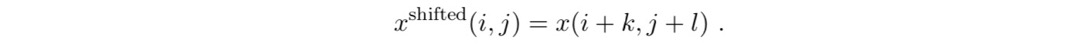In practice, boundary conditions should be considered for pixels (i, j) such that (i + k, j + l) not equal to [0, n1-1] x [0, n2-1].

A typical example is to consider periodical boundary conditions such that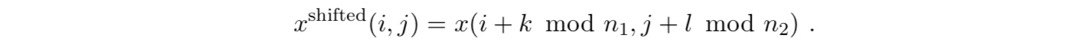Create in imshift function implementing the shifting of an image x in periodical boundary, such as the following image(b) Shifted in the direction (k,l) by (+100,-50):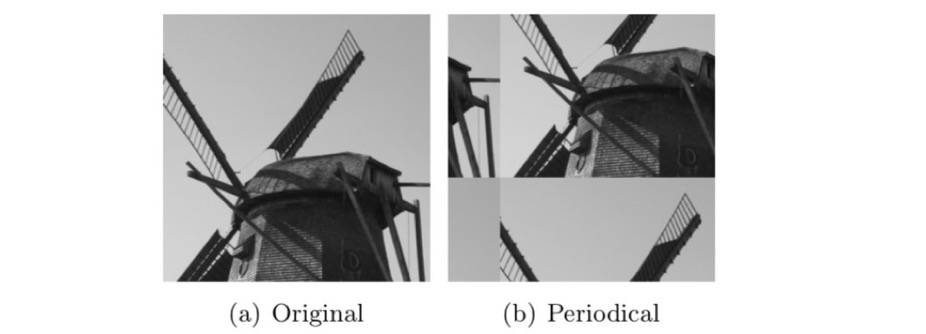Hint: First write it using loops, and next try to get rid of the loops.

import numpy as np
import matplotlib.pyplot as plt

def imshift(x, k, l):
'''
'''
k = k % x.shape
l = l % x.shape

xshifted_v = np.zeros(x.shape)
xshifted = np.zeros(x.shape)

if k != 0:
xshifted_v[:k, :] = x[-k:, :]
xshifted_v[k:, :] = x[:-k, :]
else:
xshifted_v = x

if l != 0:
xshifted[:, :l] = xshifted_v[:, -l:]
xshifted[:, l:] = xshifted_v[:, :-l]
else:
xshifted = xshifted_v

return xshifted

#Sample call and Plotting code
#“lake.png” and "windmill.png"
'''
'''
img1_s = imshift(img1, 100, 50)
img2_s = imshift(img2, 100, 50)
plt.imshow(img1_s, cmap='gray')
plt.show()
plt.imshow(img2_s, cmap='gray')
plt.show()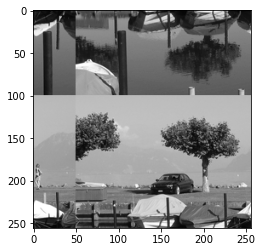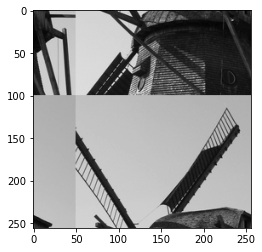Check on x = windmill.png and y = lake.png, if this operation is linear, i.e.,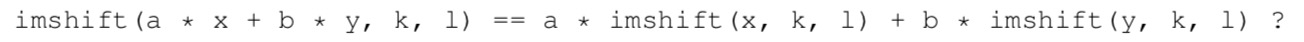After shifting the image in the direction (k, l), shift it back in the direction ( k, l). Interpret the results. Which shift is one-to-one?

'''
'''
# verifying linearity
img_12s = imshift(img1/3*2 + img2/2, 100, 50)
plt.subplot(1,2,1)
plt.imshow(img_12s, cmap='gray')
plt.subplot(1,2,2)
plt.imshow(img1_s/3*2 + img2_s/2, cmap='gray')
plt.show()

# one-to-one shift
img_to = imshift(img1, 100, 50)
img_back = imshift(img_to, -100, -50)
plt.title("one-to-one")
plt.subplot(1, 2, 1)
plt.imshow(img1, cmap='gray')
plt.subplot(1, 2, 2)
plt.imshow(img_back, cmap='gray')
plt.show()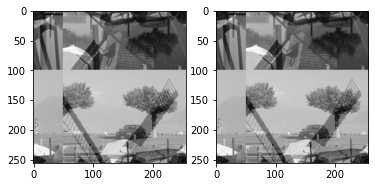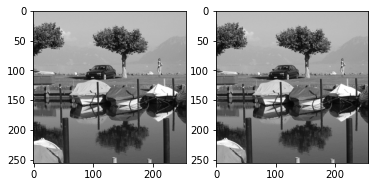## Problem 2 Convolution

In this problem, we intend to explore and implement 2D convolution.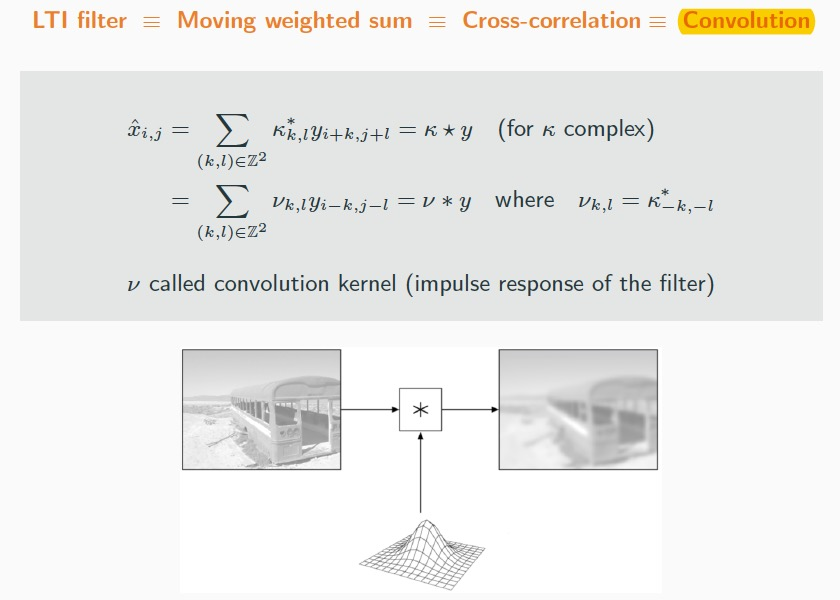First, Create imkernel function that produces a function handle nu implementing a convolution kernel functions on the finite support (-s1, s1)x(-s2, s2). In this case, we specifies the ’gaussian’ kernel as following.

Create imconvolve_naive function that performs(except around boundaries) the convolution between x (image) and v (kernel) with four nested loops [ The outer 2 loops for looping over the image, inner 2 loops for looping over the kernel].

Create imconvolve_spatial function that performs the convolution between x (image) and v (kernel) including around boundaries. The spatial method intend to create a stacked-up image, which is a 3D matrix with dimension [ image_height x image_width x total_kernel_number]. The final code should read with only two loops.

The idea is to switch the inner loops with the outer loops, and then make use of imshift to eliminate the need to use the outter loop to go over the entire image, but use shift function to shift the image along the inner loop and store the each result into your 3D matrix. With the stacked-up matrix, you can perform convolution calculation with ease. on the 3D matrix.

Write a script test_imconvolve function that compares the results and the execution times of imconvolve_naive and imconvolve_spatial, give comment on the execution times of two methods. You should have similar result like: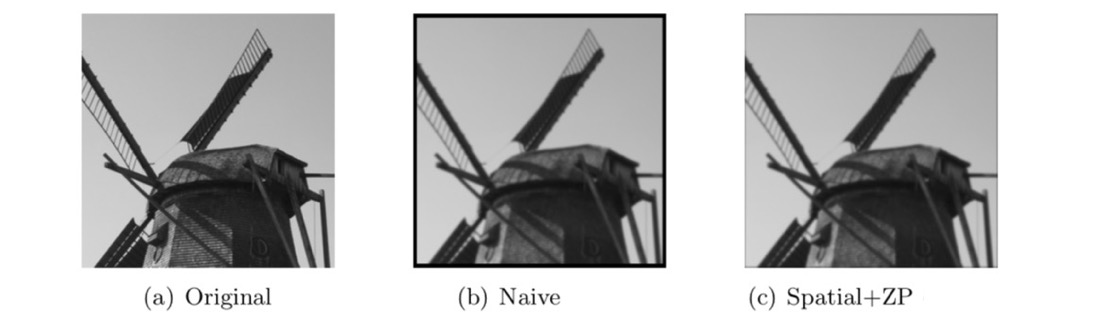import numpy as np
import matplotlib.pyplot as plt
import time

def imshift(x, k, l):
xshifted_v = np.zeros(x.shape)
xshifted = np.zeros(x.shape)
if k != 0:
if k < 0:
xshifted_v[:k, :] = x[-k:, :]
if k > 0:
xshifted_v[k:, :] = x[:-k, :]
else:
xshifted_v = x

if l != 0:
if l < 0:
xshifted[:, :l] = xshifted_v[:, -l:]
if l > 0:
xshifted[:, l:] = xshifted_v[:, :-l]
else:
xshifted = xshifted_v

return xshifted

def imkernel(tau, s1, s2):
"""
The kernel (window) function already, you can use that function to generate your kernel.

Note: the function is slightly difference than just a matrix. As you can see it returned a
lambda function object. You need to assign location of the kernel, then it will return
specified value in that location of the kernel.

For example, we want a 3x3 window, (note: in this function, we said the center point to be
location (0,0), so s1 is the absolution distance to the center point, for example: s1 means
from -1 to 1):

nu = imkernel(tau,s1,s1);   #<------- generated a 3x3 window funtion
nu(-1,-1)  #<--------- this will return the top left corner value of the kernel
nu(0,0)  #<--------- this will return the center value of the kernel

"""
w = lambda i, j: np.exp(-(i ** 2 + j ** 2) / (2 * tau ** 2))
# normalization
i, j = np.mgrid[-s1:s1+1, -s2:s2+1]  # revised
Z = np.sum(w(i, j))
nu = lambda i, j: w(i, j) / Z * (np.absolute(i) <= s1 and np.absolute(j) <= s2)  # revised
return nu  # why not return the whole kernel

# Create imconvolve_naive function,
def imconvolve_naive(x, nu, s1, s2):
(n1, n2) = x.shape
# (s1, s2) = win_size
xconv = np.zeros((n1, n2))
'''
'''
# outer loop to go through img
for i in range(n1-2*s1):
for j in range(n2-2*s2):
# inner loop to go through kernel
for ii in range(-s1,s1+1):
for jj in range(-s2,s2+1):
xconv[i+s1, j+s2] += x[i+s1+ii, j+s2+jj] * nu(ii, jj)
return xconv

# Create imconvolve_spatial function
def imconvolve_spatial(x, nu, s1, s2):
'''
'''
# generate kernel and 3D matrix
gaussian_kernel = np.zeros([2*s1+1, 2*s2+1])
td_matrix = np.zeros([x.shape, x.shape, (2*s1+1)*(2*s2+1)])
for i in range(0, 2*s1+1):
for j in range(0, 2*s2+1):
gaussian_kernel[i, j] = nu(i-s1, j-s2)
td_matrix[:, :, (2*s2+1)*i+j] = imshift(x, i-s1, j-s2)

# reshape kernel to 1 dimension
gaussian_kernel = gaussian_kernel.reshape(-1, 1)

# matrix product
xconv = td_matrix.dot(gaussian_kernel)

return xconv

# Create test_imconvolve function
def test_imconvolve(x, nu, s1, s2):
t = time.time()
xconv_s = imconvolve_spatial(x, nu, s1, s2)
ts = time.time() - t

t = time.time()
xconv_n = imconvolve_naive(x, nu, s1, s2)
tn = time.time() - t
return xconv_s, xconv_n, ts, tn

# Sample call and Plotting code
tau = 1
s1 = 4
s2 = 4

'''
'''
# read image and set kernel
nu = imkernel(tau, s1, s2)

# test call

(img_conv_spatial, img_conv_naive, ts, tn) = test_imconvolve(img_1, nu, s1, s2)

# ploting
print("spatial_conv_time: ", ts, "\nnaive_conv_time: ", tn)
plt.imshow(img_conv_spatial, cmap='gray')
plt.title("Spatial")
plt.show()
plt.imshow(img_conv_naive, cmap='gray')
plt.title("Naive")
plt.show()

spatial_conv_time:  0.04901123046875
naive_conv_time:  29.49830460548401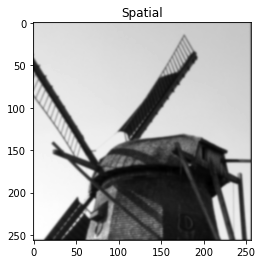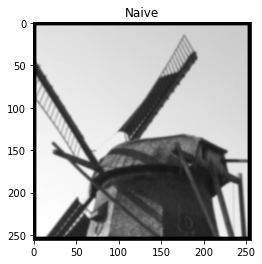## Problem 3: Order-statistic filtering

Order-statistic filters (OSF) are local filters that are only based on the ranking of pixel values inside a sliding window.

1. Create in imstack(img,s1,s2) function that creates a stack xstack of size n1 ×n2 ×s, which s = (2s1 +1)(2s2 +1) from the n1 ×n2 image x, such that xstack(i,j,:) contains all the values of x in the neighborhood (−s1, s1) × (−s2, s2). This function should take into account the four possible boundary conditions.

Hint: you can use imshift, which we implemented in assignment 1, and only two loops for −s1 <= k <= s1 and −s2<= l<= s2.

2. Create in imosf() function
function imosf(x, type, s1, s2) that implements order-statistic filters, returns xosf. imosf should first call imstack, next sort the entries of the stack with respect to the third dimension, and create the suitable output xosf according to the string type as follows:

• 'median': select the median value,

• 'erode': select the min value,

• 'dilate': select the max value,

• 'trimmed': take the mean after excluding at least 25% of the extreme values on each side.

3. Create in imopening() and imclosing() function that performs the opening and closing by the means of OSF filters.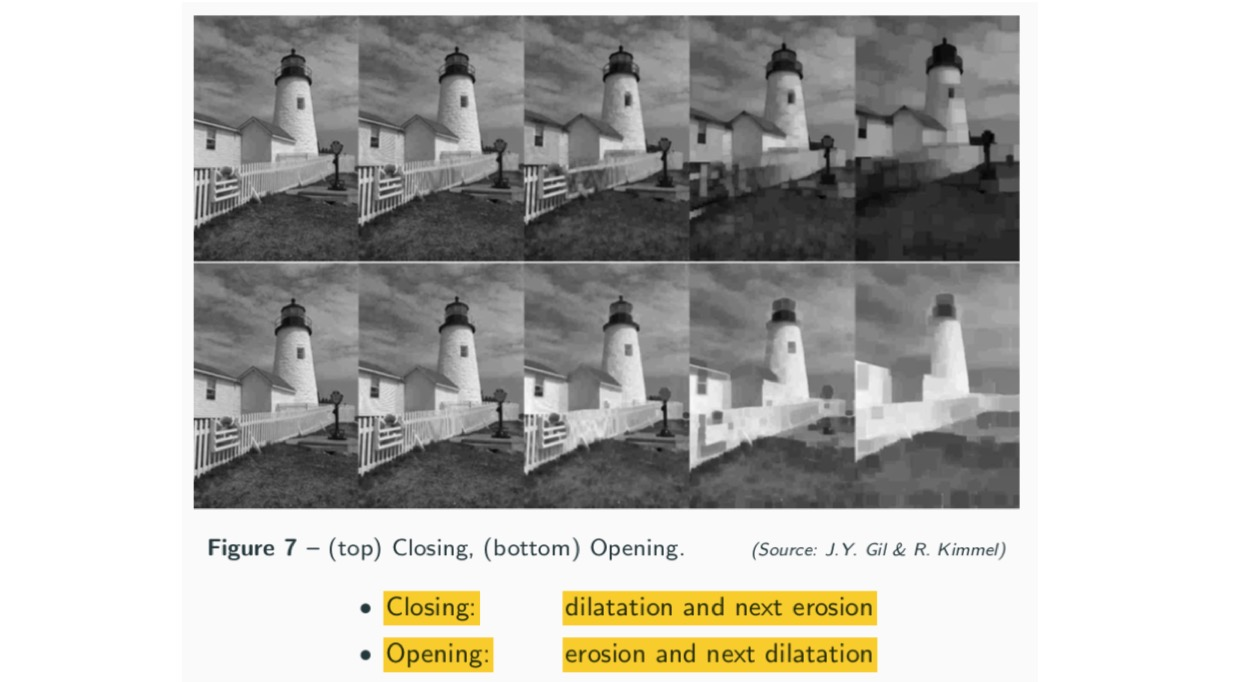4. Load castle.png. Write a script to test imosf() that loads the image x = castle and create a corrupted version of image x with 10% of impulse noise (salt and pepper)

Apply your OSF filters and zoom on the results to check that your results are consistent with the following ones: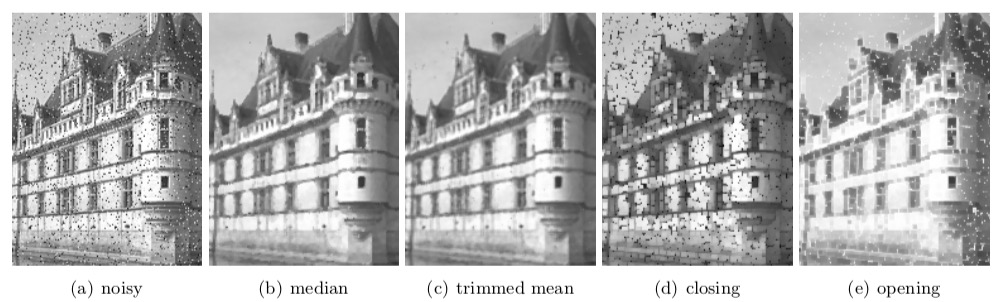Note: Typo on image, (d) -- opening , (e) -- closing

import numpy as np
import matplotlib.pyplot as plt
from skimage import util

def imshift(x, k, l):
# deal with boundaries, by replicating edges
xshifted = x_padded[(abs(k) - k):(abs(k) - k + x.shape), (abs(l) - l):(abs(l) - l + x.shape)]
return xshifted

def imstack(img, s1, s2):
# generate 3D matrix
img_stack = np.zeros([img.shape, img.shape, (2 * s1 + 1) * (2 * s2 + 1)])
for i in range(0, 2 * s1 + 1):
for j in range(0, 2 * s2 + 1):
img_stack[:, :, (2 * s2 + 1) * i + j] = imshift(img, i - s1, j - s2)
# sort
img_stack = np.sort(img_stack)  # quicksort
return img_stack

def imOSF(img, s1, s2, type='median'):
assert type == 'median' or \
type == 'erode' or \
type == 'dilate' or \
type == 'trimmed', 'no such type'
img_stack = imstack(img, s1, s2)
if type == 'median':  # select the median
img_osf = img_stack[:, :, int(img_stack.shape / 2)]
elif type == 'erode':  # select min
img_osf = img_stack[:, :, 0]
elif type == 'dilate':  # select max
img_osf = img_stack[:, :, img_stack.shape - 1]
elif type == 'trimmed':  # take mean after excluding extreme values
img_osf = np.mean(img_stack[:, :, int(img_stack.shape / 4):int(img_stack.shape / 4 * 3)], axis=2)
return img_osf

def imopening(img, s1, s2):
img_opening = imOSF(img, s1, s2, type='erode')
img_opening = imOSF(img_opening, s1, s2, type='dilate')
return img_opening

def imclosing(img, s1, s2):
img_closing = imOSF(img, s1, s2, type='dilate')
img_closing = imOSF(img_closing, s1, s2, type='erode')
return img_closing

# Import image
oimg = imread("peppers.png")  # no castle.png
plt.title("(a)original")
plt.imshow(oimg, cmap='gray')
plt.show()

# Set window size
s1 = 1
s2 = 1

nimg = util.random_noise(oimg, mode='s&p', amount=0.1)
plt.title("(b)noisy")
plt.imshow(nimg, cmap='gray')
plt.show()

# median
osfimg_m = imOSF(oimg, s1, s2, 'median')
plt.title("(c)median")
plt.imshow(osfimg_m, cmap='gray')
plt.show()

# trimmed
osfimg_t = imOSF(oimg, s1, s2, 'trimmed')
plt.title("(d)trimmed")
plt.imshow(osfimg_t, cmap='gray')
plt.show()

# opening
img_opening = imopening(nimg, s1, s2)
plt.title("(e)opening")
plt.imshow(img_opening, cmap='gray')
plt.show()

# closing
img_closing = imclosing(nimg, s1, s2)
plt.title("(f)closing")
plt.imshow(img_closing, cmap='gray')
plt.show()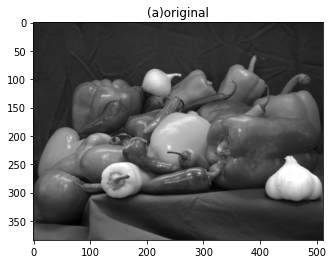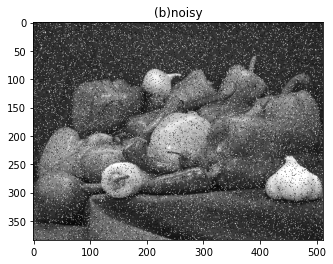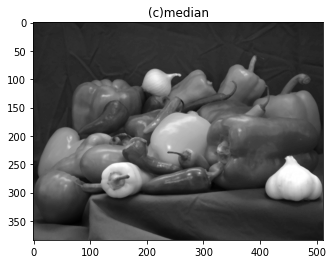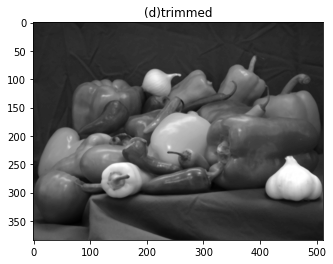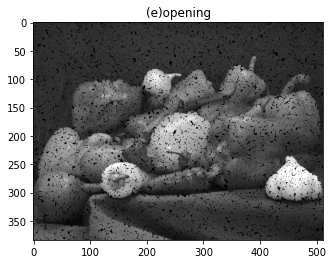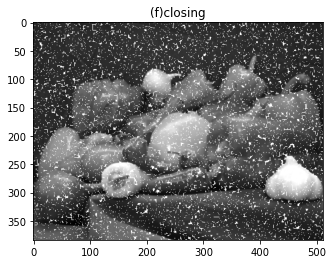Q.E.D.

To baldly -_- go no man has gone before